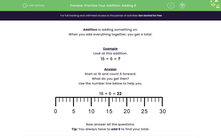### Comprehensive & curriculum aligned

In this worksheet, students complete addition problems by adding the number 6.Key stage:  KS 1

Curriculum topic:   Number: Addition and Subtraction

Curriculum subtopic:   Add and Subtract to 20

Difficulty level:#### Worksheet Overview

When you add everything together, you get a total.

Example

16 + 6 = ?

Start at 16 and count 6 forward.

What do you get then?

16 + 6 = 22